A method for 3D-reconstruction of volcanic bomb trajectories
Kelfoun et al. (2020), Bulletin of Volcanology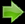Download codes, images and movies

The codes are written in Matlab and compatible with the free software Octave.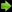detect_bombs.m The first code is used to detect the bombs on each image. It saves the coordinates of the bombs and their luminosity in the « bombs » directory. Modify the code at line 16 to compute bomb positions of cam42. uses: bpass.m and pkfnd.m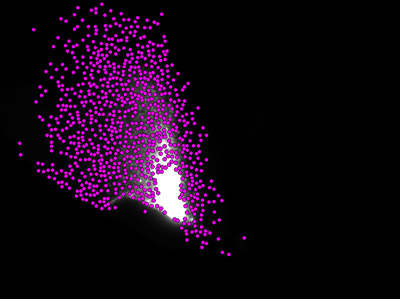detect_trajectories.m Once all the images have been analysed, detect_trajectories.m identifies the same bomb on each image of one camera to reconstruct its trajectory. Run the code for cam49 by changing line 24.The program then analyses the trajectories obtained to check if they are close enough from a parabola (lines 193-242). For the example, only bombs detected on image 60 are analysed. Change line 32 to treat all the images. Another code – not given – merges all the similar trajectories calculated form various initial images. uses recursively: detect_next_bomb.m see also the movie '2D trajectories.avi' (16 Mo)camera_orientation_with_relief.m Allows calculating manually the orientations of the cameras. A DEM must be used for the topographic projection. A very small area of the DEM used (Favalli et al., 2009) can be downloaded here: Codes (150 ko). uses: draw_relief_on_image.m, correct_distorsions, cam42.m, cam49.m, XYZ_to_ccd and ccd_to_3D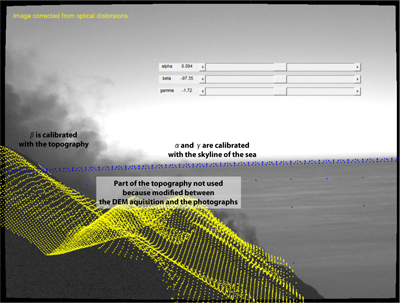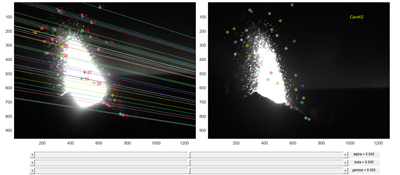computes_relative_orientation_by_epipolar.m This program uses the positions of bombs on camera 42. Its then computes the corresponding epipolar lines on image 49. For the article, we have used an automatic version of the code shown, by exploring the values of the three angles in a given range (+/- 0.5°) and recording these for which D, the sum of the distances points/epipolar lines to the square (see line 137), is minimum. uses: correct_distorsions.m, cam42.m and cam49.mSeeks_matching_trajectory_from_epipolar.m Choses each trajectories detected for camera 42 (here only the bombs visible on image 60) and seeks the same trajectory on camera 49 by using the proximity of bombs with the epipolar line. Change lines to see other trajectories and other frames. This demo program is a simplified version of the code used (for clarity and to speed up the code) and is designed as a tutorial. For some bombs, several trajectories can match (mostly for short trajectories). For the article, we have used a combination of this code with reconstruction_3D.m (see next) that calculates the trajectory in 3D. Thus, other criteria can be used to validate the matching: the hyperbola shape, a realistic value of the gravity by fitting with a ballistic model and a parabola starting from the vent. uses: correct_distorsions.m, cam42.m and cam49.m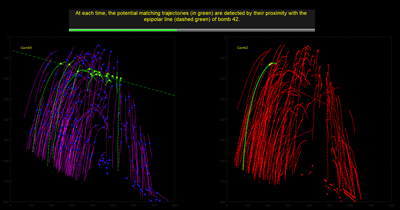see the animated gif (8Mo)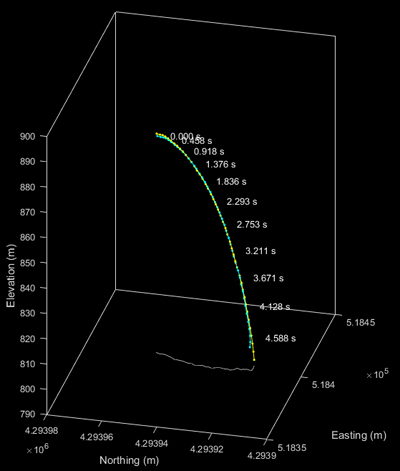reconstruction_3D.m ses the matching trajectories on cameras 42 and 49 to calculate the bomb trajectory in 3D. Here only the matching trajectory of the figure of Seeks_matching_trajectory_from_epipolar.m is used. In the article, cam42 is called camera 2 and cam49 is called camera 1.XYZ_to_ccd.m Used to project a point in space onto the ccd sensor of the camera according to its position and its orientation.ccd_to_3D.m Used to calculate the position of a point of the ccd sensor in space and then to calculate the equation of the line where this point can be in space (lines L1 or L2 of Fig. 1 of the article).correct_distorsions Uses the lens distortions of the cameras to correct the positions of points recorded on the ccd sensor.